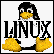## binary andhexadecimal notation

### .. binary ..

Arithmetic rules apply, rather straightforwardly, so that, for example, 1+0=1 and 0+1=1, etc. The only things you need to remember are..

• The only values used are 0 and 1.
• The column values from left to right are powers of 2,
that is, .., 32, 16, 8, 4, 2, 1.
• For addition, 1 + 1 = 0, carry 1

### .. binary example..

To represent a decimal number in binary, just start subtraction the largest powers of two you can think of. As an example, a decimal number such as 94, would contain 64 as the largest power of two (the next largest is 128). Do the following ..

Since 64 is the largest power of two which is less than 94..
Write a 1 in the 64's column, subtract 64 from 94.

100,0000-bin, and 30 left over

Since 32 is too big, subtract 16 as the next largest power of two..
Write a 1 in the 16's column, subtract 16 from 30.

101,0000-bin, and 14 left over

Since 8 will fit, subtract 8 as the next largest power of two..
Write a 1 in the 8's column, subtract 8 from 14.

101,1000-bin, and 6 left over

Since 4 is the largest power of two which is less than 6..
Write a 1 in the 4's column, subtract 4 from 6.

101,1100-bin, and 2 left over

Since 2 is a power of two..
Write a 1 in the 2's column, subtract 2 from 2.

101,1110-bin, and 0 left over

To add two binary numbers, for example the numbers 1001 and 0111 just line them up, start adding ones and doing carries..

```carries        111
first num      1001
second num     0111
----
result        10000
```

Arithmetic rules, again, apply.

• The following values are used, representing zero through 15 decimal
0 1 2 3 4 5 6 7 8 9 A B C D E F
• The column values from left to right are powers of 16,
that is, .., 256, 16, 1.
Hex numbers greater than 2 digits are not generally used.
• For addition, F + 1 = 0, carry 1

### .. hex example..

Hexadecimal is not used to represent decimal numbers, but is used almost exclusively as a shorthand for representing binary numbers. Look at the [hex] page for why this is so.

To add two hex numbers, say 3F and 9A, just add the individual columns in decimal, and keep subtracting quantities of 16 (which carries to the next column).

```
carries           1
first num         3F
second num        9A
----------
sum           (3+9)(A+F)
or...             D9
```HOST: Outflux.net, http://www.Outflux.net
URL: http://jnocook.net/user/column.htm

17